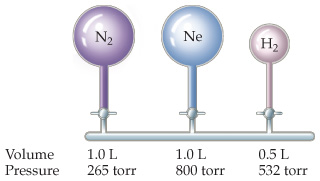# Problem: Consider the arrangement of bulbs shown in the drawing. Each of the bulbs contains a gas at the pressure shown.What is the pressure of the system when all the stopcocks are opened, assuming that the temperature remains constant? (We can neglect the volume of the capillary tubing connecting the bulbs.)

🤓 Based on our data, we think this question is relevant for Professor Cruz's class at USF.

###### FREE Expert Solution

We are asked to determine the pressure of the system when all the stopcocks are opened, assuming that the temperature remains constant.According to Boyle’s Law, an inverse relationship exists between pressure and volume assuming the number of molecules (n) and the temperature (T) are both constant.

The relationship for Boyle’s Law can be expressed as follows:

where:

P1 and V1 are the initial pressure and volume values

P2 and V2 are the values of the pressure and volume of the gas after the change.###### Problem Details

Consider the arrangement of bulbs shown in the drawing. Each of the bulbs contains a gas at the pressure shown.

What is the pressure of the system when all the stopcocks are opened, assuming that the temperature remains constant? (We can neglect the volume of the capillary tubing connecting the bulbs.)